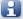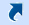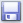Curled tiles editor
 22.1. Curled tiles editor allows you to set the type of curled tiles from the available list and specify its extra parameters (dimensions). The list of types is on the right, this list is non-editable for the user. It will be added with new types in subsequent versions of the program on regular bases. Tile shape in the end is determined by the dimensions of the tiles defined in the materials datebase, by the type of tile and additional options (if needed).The features of the types. The 'left' and the 'right' is determined by 'prow' (protruding part) of the curled tiles. The derection of the 'prow' determines the type of the curled tale. It`s important to notice that there is some confusion in the catalogues for this term, so we proceed from the top of the protruding part (if there is any). For rectangles with bevels and right-angle triangles - right is the one in which the upper right corner is not cut. 1. Type 'Parallelogram' (chevron). The dimensions of the parallelogram in the tile is considered to be the height and base of the chevron (not the width), so the width is actually larger than the specified size. 2. Type 'Hexagon right'. The dimensions of a regular hexagon must have a ratio w=h/ (v3/2) (w - width, h - height). 3. Type 'Octagon right'. The dimensions of a regular octagon (the octagon) should be equal (w=h). 4. Type 'Fan'. In this type there is a complex dependence between the dimensions. Therefore, when you define additional parameters you have to put the parameter P as equal to zero (P=0 cm.), the system calculates it automatically. And only when you have decided on the first two additional parameters, enter the parameter P (in fact it is determined by the distance between the two cut radii of the left and right and these radii are equal to the additional parameter R).   Additional default values. 1. Rhombus with bevels of corners. The size of the bevel is R = 2 cm. 2. Rhombus with radius cuts. Cut radius R = 0.75 cm. 3. Isosceles triangle. The radius of the curvature of the corners is R = 0 cm. 4. Parallelogram (chevron) left and right. The size of the bevel R is determined by the angle of inclination of 60 degrees. 5. Trapezium. The size of the bevel R is determined by the angle of inclination of 60 degrees. 6. Pentagon. The height of the bevel R is equal to half the height of the tile. 7. Irregular hexagon. The height of the vertical side R is equal to half the height of the tile. 8. Irregular octagon. The size of the bevels is R = 2 cm. 9. Fan. The radius of curvature R is equal to half the width of the tile. The height of the top part V is equal to the radius of curvature R. The tail width P is calculated automatically, but is an editable parameter. 10. Rectangle with two bevels right and left. The dimensions of the bevels are R = 2 cm and V = 2 cm. 11. Rectangle with bevels. The dimensions of the bevels are R = 2 cm and V = 2 cm. 12. Rectangle corners with rounded edges. Radius of curvatures R = 2 cm. 13. Rectangle corners with radius cuts. Cutting radius R = 2 cm. 14. Rectangle radius cut is in the center. The radius of the cut R is 1/4 of the smaller side. 15. Rectangle radius cut of the corner. Radius cut R = 2 cm. 16. Rectangle radius cut on the sides. The radius of the cut R is equal to the longer side. 17. Cross. The width of the blade of the cross R is equal to half of the smaller side. If the optional parameter cannot be set by default due to the overall dimensions, the minimum possible value will be set.   After having entered the required additional values, please, click 'Apply' button. The tile will be changed according to the entered dimensions. The dimensions of tiles, as well as the tile types are not added into memory this way. In order to save the dimensions and types of tiles, please, click 'Save'. The texture of the tiles will be generated and all data will be saved and the window will be closed. The 'Cancel' button will cancel all actions and close the window.   At the stage of 'fitting' the texture on the tile, you can use the buttons at the bottom of the window. They serve to stretch or scale the texture. Each press of the button changes the current position / scale of the texture by 1 pixel. The buttonis for viewing the original texture. The buttonserves to restore the original texture. The texture will be loaded into the current tile on the screen. Using the button, you can overwrite the original texture with the current texture.   The original textures will be saved automatically in the tile directory when creating a curled tile and may be needed to change the tile type. The fact is that if you save the tile as a curled one, the texture changes under the outline of the tile contour.